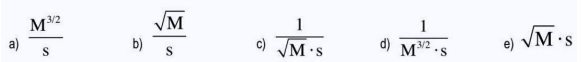# Problem: If the rate for the following reaction is found to be the following: Rate = k [Cl2]3/2 • [HCCl3]. What are the units for the rate constant, k? Cl2 (g) + HCCl3 (g)       →      HCl (g) + CCl 4 (g)

###### FREE Expert Solution
91% (495 ratings)
###### Problem Details

If the rate for the following reaction is found to be the following:

Rate = k [Cl2]3/2 • [HCCl3]. What are the units for the rate constant, k?

Cl2 (g) + HCCl3 (g)       →      HCl (g) + CCl 4 (g)